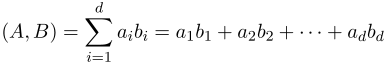Day 1, Problem 1 - Inner Product

The inner product (a.k.a. dot product) of two d-dimensional vectors A = [a1, a2, …, ad] and B = [b1, b2, …, bd] is equal to the sum of products of their corresponding components. Specifically:$\displaystyle (A,B)=\sum_{i=1}^{d}a_ib_i=a_1b_1+a_2b_2+\cdots +a_db_d$

Given n such d-dimensional vectors, x1, …, xn, Little Meow-Meow would like to know if there exists two vectors whose inner product is a multiple of k. Please help her solve this problem.

Input Format

The first line of input contains 3 positive integers n, d, and k, respectively representing the number of vectors, the number of dimensions, and the number of which a inner product could be a multiple.
The next n lines each contains d nonnegative integers. On the i-th of these lines, the j-th integer represents xi,j, the j-th component of vector xi.

Output Format

Output two integers, separated by a space.
If there exists two vectors xp and xq whose inner product is an integer multiple of k, then output their indices p and q (p < q). If there are multiple answers, output any one of them.
If an answer does not exist, then output two -1's separated by a space.

3 5 2
1 0 1 0 1
1 1 0 1 0
0 1 0 1 1

2 3

(x1, x2) = 1
(x1, x3) = 1
(x2, x3) = 2

Constraints

Test Casendkxi,j
12202≤ 10
25202≤ 10
310203≤ 10
420202≤ 100
550203≤ 100
650502≤ 1000
750503≤ 3000000
880802≤ 2000000
91001003≤ 3000000
105001003≤ 3000000
1110001002≤ 2000000
1210001003≤ 3000000
13100001002< 10
14100001003< 10
15150001002< 10
16180001002< 10
17200001002< 10
1850000303< 10
1980000303< 10
20100000303< 10

Point Value: 20 (partial)
Time Limit: 5.00s
Memory Limit: 256M

Languages Allowed:
C++03, PAS, C, HASK, ASM, RUBY, PYTH2, JAVA, PHP, SCM, CAML, PERL, C#, C++11, PYTH3

• (0/0)
I think many solutions will fail on this test:

5 3 3
1 1 1
1 1 1
1 1 1
1 1 1
0 0 1

• (1/0)
I'm pretty that the answers for the data are unique. However, if you seriously suspect that a custom grader is needed, let me know.

• (0/0)
Nick Wu wrote a program that received WA (i.e. his program claimed that a solution exists and this solution is different from the expected solution). This means that multiple solutions are possible?

• (0/0)
I think he guessed after running out of time.

• (0/0)
To close the loop, a custom grader is required for the given test data.

• (0/0)